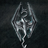首页   注册   登录
V2EX = way to explore
V2EX 是一个关于分享和探索的地方

Learn Python the Hard Way
Python 学习手册Python CookbookPython 基础教程Python Sites
PyPI - Python Package Index
http://www.simple-is-better.com/
http://diveintopython.org/toc/index.html
Pocoo

PyPy
Celery
Jinja2
gevent
pyenv
virtualenv
Stackless Python
Beautiful Soup

Green Unicorn
Sentry
Shovel
Pyflakes
pytest
Python 编程
pep8 Checker
Styles
PEP 8
Code Style from The Hitchhiker's Guide
V2EX  ›  Python

# 遇到一个对应计算的问题

love642285 · 118 天前 · 644 次点击

B = np.random.randint(0,q,(m,n))
for i in range(0,N):
exec("s%s=np.random.randint(0,q,(n,1))"%i)
for i in range(0,N):
exec("e%s=np.random.randint(0,q,(m,1))"%i)
for i in range(0,N):
exec("b%s=np.dot(B,s%s)+e%s"%i)

exec("b%s=np.dot(B,s%s)+e%s"%i)
TypeError: not enough arguments for format string
3 回复  |  直到 2019-08-13 21:16:54 +08:001 cherbim   118 天前 not enough arguments for format string这个圈起来，期末考试要考，话说你知道这个报错啥意思么2 cherbim   118 天前 最后一行你要传递三个参数，虽然三个参数相同，那也不能省略3 necomancer   118 天前 B = np.random.randint(0,q,(m, n))s = np.random.randint(0,q,(N, n))e = np.random.randint(0,q,(N, m))b = np.einsum('ij,...j->...i', B, s) + eb -> (N, m)